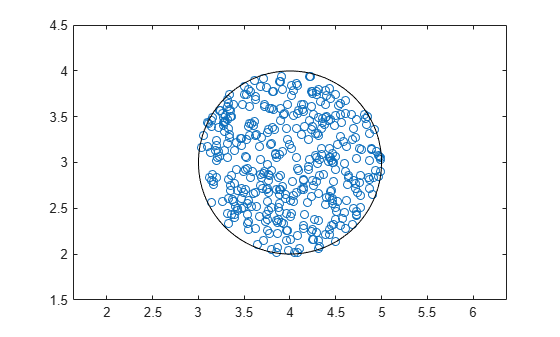# ucomplex

Create uncertain complex parameter

## Syntax

```A = ucomplex('NAME',nominalvalue)
A = ucomplex('NAME',nominalvalue,'Property1',Value1,...
'Property2',Value2,...)
```

## Description

An uncertain complex parameter is used to represent a complex number whose value is uncertain. Uncertain complex parameters have a name (the `Name` property), and a nominal value (the `NominalValue` property).

The uncertainty (potential deviation from the nominal value) is described in two different manners:

• `Radius` (radius of disc centered at `NominalValue`)

• `Percentage` (disc size is percentage of magnitude of `NominalValue`)

The `Mode` property determines which description remains invariant if the `NominalValue` is changed (the other is derived). The default `Mode` is` 'Radius'` and the default radius is 1.

Property/Value pairs can also be specified at creation. For instance,

```B = ucomplex('B',6-j,'Percentage',25) ```

sets the nominal value to `6-j`, the percentage uncertainty to `25` and, implicitly, the `Mode` to `'Percentage'`.

## Examples

collapse all

Compute 400 random samples of an uncertain complex parameter and visualize them in a plot.

Create an uncertain complex parameter with internal name `A`.

`A = ucomplex('A',4+3*j)`
```A = Uncertain complex parameter "A" with nominal value 4+3i and radius 1. ```

The uncertain parameter's possible values are a complex disc of radius 1, centered at 4 + 3_j_. The value of `A.percentage` is 20 (radius is 1/5 of the magnitude of the nominal value).

You can visualize the uncertain complex parameter by sampling and plotting the data.

```sa = usample(A,400); w = linspace(0,2*pi,200); circ = sin(w) + j*cos(w); rc = real(A.NominalValue+circ); ic = imag(A.NominalValue+circ); plot(real(sa(:)),imag(sa(:)),'o',rc,ic,'k-') xlim([2.5 5.5]) ylim([1.5 4.5]) axis equal```## Version History

Introduced before R2006a